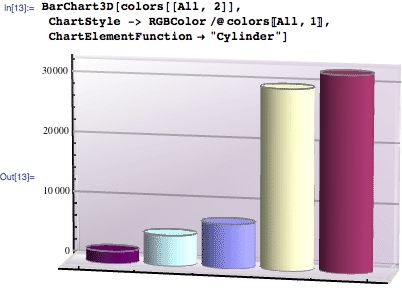Browse by Topic
Related Topics

# Interactive Angle Measurement with Mathematica

Consider the typical infographics found on the internet, many of which are only slightly less silly than this one by Jamie Schimley: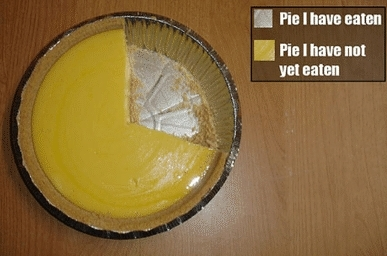If you want to regenerate a chart such as this in Mathematica using the PieChart function, you need hard data: the relative areas of the slices. You could eyeball the values and get an approximation, but since I deal with user interfaces I was immediately interested in creating one that would allow me to measure the angle of each sector of a pie chart.

The following code creates locators that can be positioned to calculate the angle of any sector. Buttons let you record the angles as you measure them, and reproduce the chart at the end. (This could be done with less code, but I wanted a more complete interface with finishing touches like disabling the Print Chart button if you haven’t measured any angles yet, and showing the current angle with a tooltip.)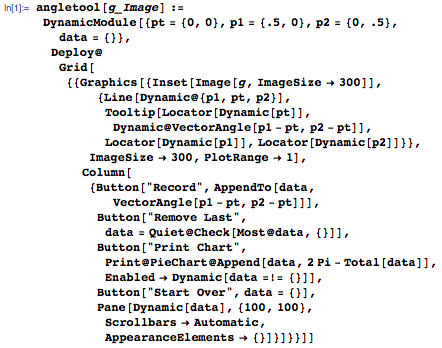This interface will appear on top of any image that is passed in as an argument. (Images from the web can be simply dragged from the browser and dropped into a Mathematica input line. They are automatically made small when combined with textual input, but the full original image is still part of the input.)

The locators initially appear at default locations: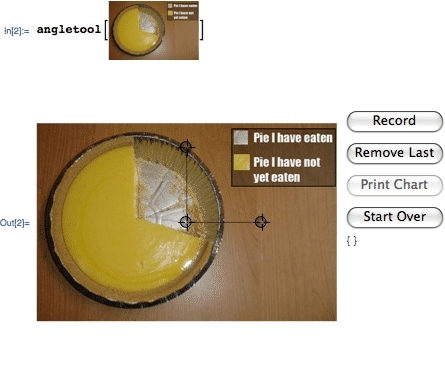But it’s a simple matter to drag them to the appropriate places in the image: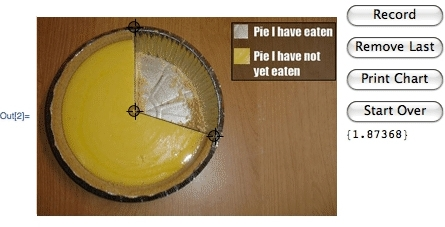Here is the chart produced by the Print Chart button.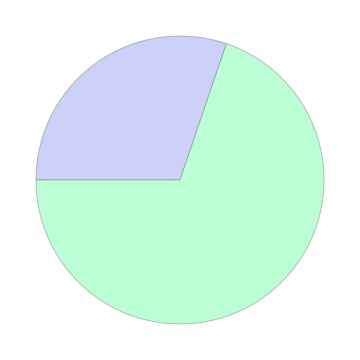A more realistic example, also harvested from the internet, includes more than just two segments: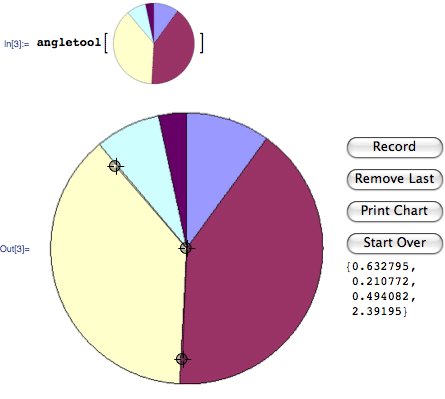The result doesn’t reproduce the colors or positions of course, but the proportions are right: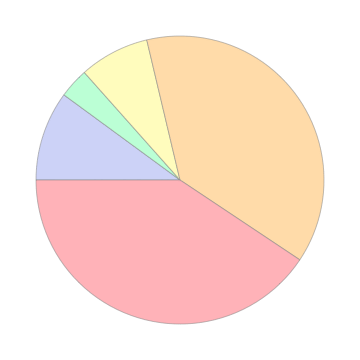A natural improvement would be some sort of algorithm where the tool would automatically detect the sector edge and perhaps snap to them. In the interest of avoiding line-detection algorithms in images, I opted to do this for polygons. The tool morphed into an angle- and length-measuring tool that snaps to an edge or a vertex of a polygon. It also detects and snaps to intersections of edges in a self-intersecting polygon.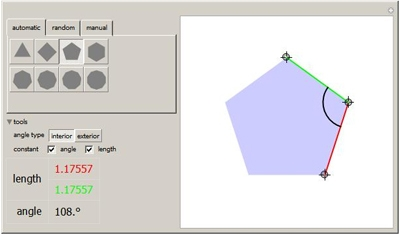This tool is an illustration not only of geometry in action but also of the development of a custom application in Mathematica. Nonetheless, it involves “manual labor” to measure each of the sectors. Mathematica is such an integrated environment that it would be a shame not to automate the process of reproducing the pie chart.

In most sensible pie charts each slice has a distinct color. That means we can measure the area of a slice by counting how many pixels have that particular color value. This is remarkably easy to do in Mathematica because images can trivially be treated as data. The Tally function reports how many of each different value occurs in a list.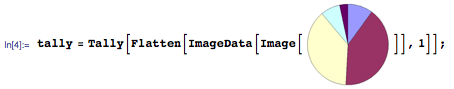The length of this list indicates how many different colors were found: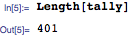Clearly there are many more colors than slices, most likely due to anti-aliased pixels that we can ignore, at least in the first approximation. Indeed, there are six colors that occur much more frequently than others (the five sector colors plus the background color).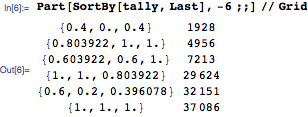The last entry is clearly the white background, so it can be eliminated. The colors and pixel counts we want are these: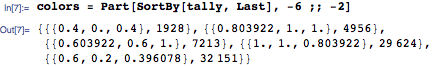We can now use the multiplicity values to recreate the pie chart. In fact, since we have the color values of the pixels we counted, we can even reproduce the color of each slice.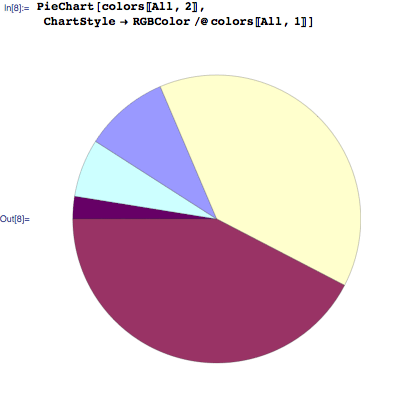Let’s package this into a function and compare the result with the original.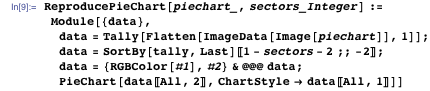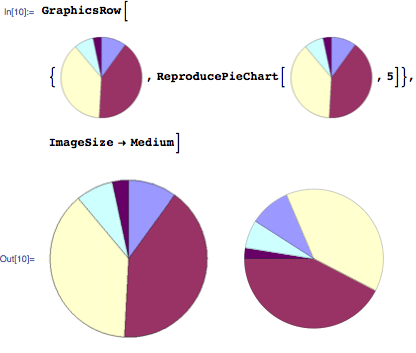For pie charts collected from the wild, some further image processing might be necessary to clean up the colors (for example, if the patches are dithered or textured, some blurring might help). Fortunately Mathematica supports a wide range of both image processing and symbolic computation features, so this can safely be left as an exercise for the reader.

Why might you want to do this in the first place? Well, for one thing, once you have the chart in Mathematica you can do neat things like click on individual segments to pull them out: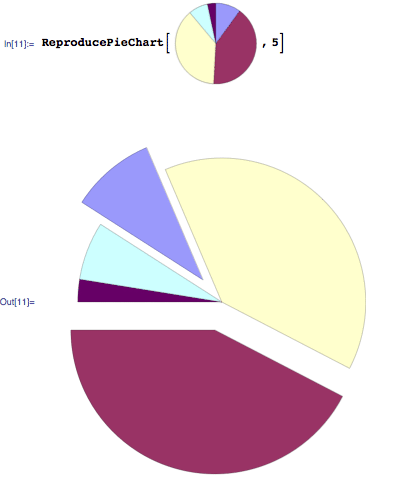Or turn it into a bar chart: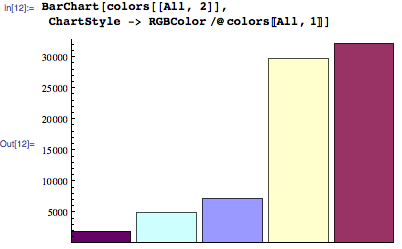Or make it pointlessly 3D: